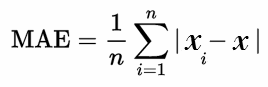# Absolute and Relative Error

## Absolute Error Definition

Absolute error is the difference between measured or inferred value and the actual value of a quantity. The absolute error is inadequate due to the fact that it does not give any details regarding the importance of the error. While measuring distances between cities kilometers apart, an error of a few centimeters is negligible and is irrelevant. Consider another case where an error of centimeters when measuring small machine parts is a very significant error. Both the errors are in the order of centimeters but the second error is more severe than the first one.

## Absolute Error Formula

If x is the actual value of a quantity and x0 is the measured value of the quantity, then the absolute error value can be calculated using the formula

Δx = x0-x.

Here, Δx is called an absolute error.

If we consider multiple measurements, then the arithmetic mean of absolute errors of individual measurements should be the final absolute error.

## Absolute Error Example

For example, 24.13 is the actual value of a quantity and 25.09 is the measure or inferred value, then the absolute error will be:

Absolute Error = 25.09 – 24.13

= 0.86

Most of the time it is sufficient to record only two decimal digits of the absolute error. Thus, it is sufficient to state that the absolute error of the approximation 4.55 to the correct value 4.538395 is 0.012.

## Relative Error

The relative error is defined as the ratio of the absolute error of the measurement to the actual measurement. Using this method we can determine the magnitude of the absolute error in terms of the actual size of the measurement. If the true measurement of the object is not known, then the relative error can be found using the measured value. The relative error gives an indication of how good measurement is relative to the size of the object being measured.

If x is the actual value of a quantity, x0 is the measured value of the quantity and Δx is the absolute error, then the relative error can be measured using the below formula.

Relative error = (x0-x)/x = (Δx)/x

An important note that relative errors are dimensionless. When writing relative errors it is usual to multiply the fractional error by 100 and express it as a percentage.

## Absolute Error and Relative Error in Numerical analysis

Numerical analysis is concerned with the methods of finding the approximate values and the absolute errors in these calculations. The absolute error gives how large the error is, while the relative error gives how large the error is relative to the correct value. In numerical calculation, error may occur due to the following reasons.

• Round off error
• Truncation error

Example 1:

Find the absolute and relative errors of the approximation 125.67 to the value 119.66.

Solution:

Absolute error = |125.67-119.66|=6.01

Relative error = |125.67-119.66|/119.66 = 0.05022

## Mean Absolute Error

The mean absolute error is the average of all absolute errors of the data collected. It is abbreviated as MAE (Mean Absolute Error). It is obtained by dividing the sum of all the absolute errors with the number of errors. The formula for MAE is:Here,

|xi – x| = absolute errors

n = number of errors